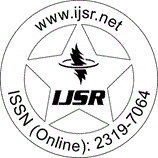International Journal of Science and Research (IJSR)
Call for Papers | Fully Refereed | Open Access | Double Blind Peer Reviewed

Research Paper | Physics Science | Sweden | Volume 10 Issue 3, March 2021

# Does Gravitation Really Depend on the Curvature of Space-Time and its Energy-Momentum?

Thomas Nordstrom PhD 

Abstract: In this paper I will investigate the postulates of Gμυ = Tμυ, i. e. the fundamental concepts of Einstein´s General Relativity. On the left side of the equation is the space-time curvature and on the right side is the energy- momentum. I will investigate if there really is equivalence between these two and also propose an alternative explanation for gravitation. Now, since the concepts of space-time curvature and energy- momentum cannot be used, we will use the concepts mass, m, and package, p, which directly represents the physical reality, where p1-n denotes the flows of packages and m denotes mass in the sense of body, system and entity. These two, m and p, also fulfil Einstein´s criterion every element of the physical reality must have a counterpart in the physical theory. So, the conclusion is that we now need to find the flows of packages in the Universe, and I will argue that: 1. Gravitation occurs when the flows of packages have an impact on the Earth and for planets, suns and galaxies, i. e. throughout the entire Universe.2. The concept flows of packages will replace the concepts of dark matter and dark energy, i. e. the flows of packages are 95 % of all matter in the Universe, while 5 % is solid matter, e. g. planets and suns.

Keywords: General Theory of Relativity, equivalence, space-time curvature, energy-momentum, gravitation, the principle of relations, flows of packages in universe, alternative theory of gravitation

Edition: Volume 10 Issue 3, March 2021,

Pages: 639 - 640